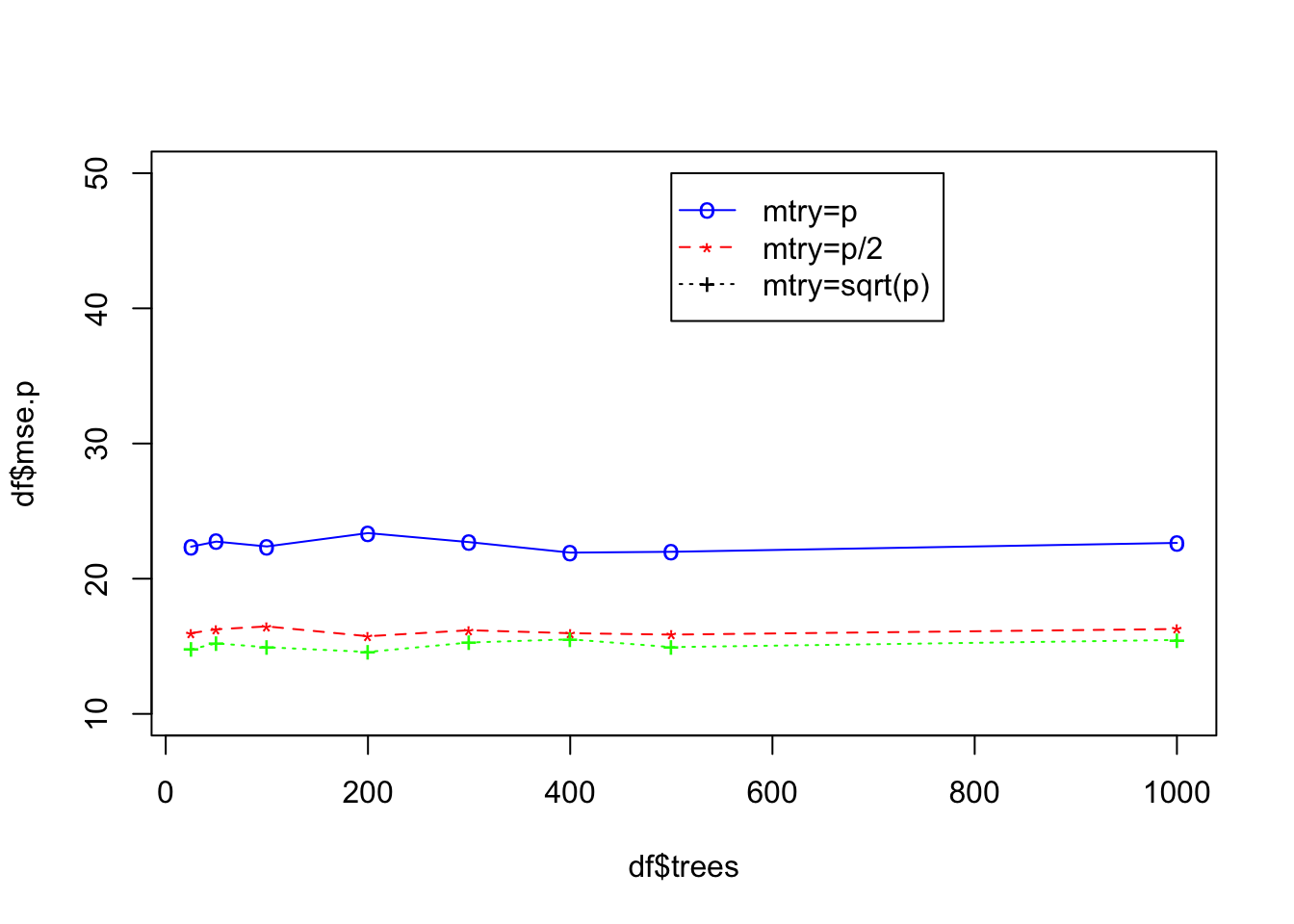ISLR Home

library(MASS)
library(randomForest)
set.seed(1)

# Creating boolean vector for train indices
train = sample(1:nrow(Boston), nrow(Boston)*0.7)

# Creating test set
boston.test = Boston[-train, "medv"]

# Setting the trees to try out
ntrees = c(25, 50, 100, 200, 300, 400, 500, 1000)
num.predictors = dim(Boston) - 1

# Creating a list of terminal nodes to try

m.tries = c(num.predictors, num.predictors/2, sqrt(num.predictors))

# Creating the vector to collect MSE
mse.p = rep(0, length(ntrees))
mse.half = rep(0, length(ntrees))
mse.sqp = rep(0, length(ntrees))

## Creating a mse dataframe to store mse

df = data.frame(mse.p, mse.half, mse.sqp)

# Looping through the array of trees and fitting a rf
for (n in 1:length(m.tries)) {

# Creating a mse vector to store mse from training for each tree
mse = rep(0, length(ntrees))

for (i in 1:length(ntrees)) {
# Fitting a random forest with i trees
rf.boston = randomForest(medv ~ .,
ntrees=ntrees[i],
data=Boston,
subset=train,
mtry=m.tries[n],
importance=TRUE)

# Predicting on the validation set
yhat.rf = predict(rf.boston, newdata=Boston[-train,])

# Calculating and adding the MSE to the mse vector
mse[i] = mean((yhat.rf-boston.test)^2)
}

# Storing the variable in the dataframe
df[n] = mse
}

df$trees = ntrees ## Plotting the MSE ## Plotting first line plot(df$trees, df$mse.p, type="o", col="blue", pch="o", lty=1, ylim=c(10,50)) ## Plotting second line points(df$trees, df$mse.half, col="red", pch="*") lines(df$trees, df$mse.half, col="red", lty=2) ## Plotting third line points(df$trees, df$mse.sqp, col="green", pch="+") lines(df$trees, df\$mse.sqp, col="green", lty=3)
legend(500,50,legend=c("mtry=p","mtry=p/2","mtry=sqrt(p)"), col=c("blue","red","black"),
pch=c("o","*","+"),lty=c(1,2,3), ncol=1)